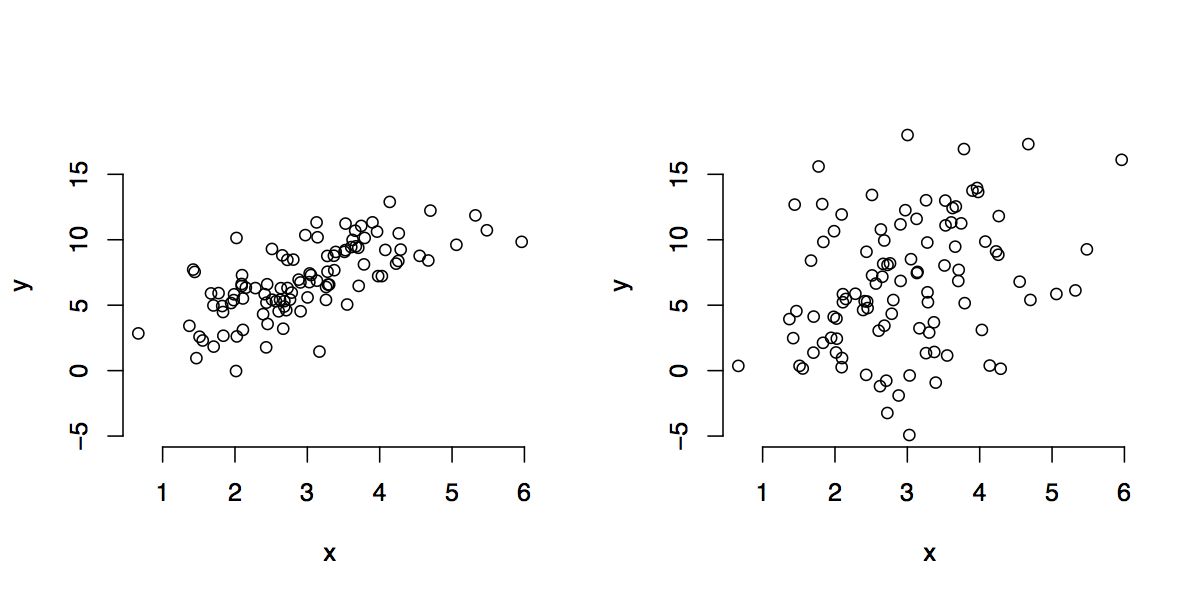28 Jul 2015

# Objective

This exercise is designed to show the students that they already have the tools they need for inference in regression models.

# Exercise

## Setup

Consider the following plots (call the left panel A and the right panel B).## Questions

1. Draw the best linear model to fit each data set.
2. In which model are you more confident? Why?
3. How might you estimate the variance of the random error terms, and how does this connect to question 2? How many degrees of freedom are used for this estimate?
4. Finally, guess what distribution the estimates of slope and intercept would follow (after appropriate centering and scaling).